# Numerical Methods For Digital Call Option Valuation

Apr. 24, 2015 2:55 PM ET
Please Note: Blog posts are not selected, edited or screened by Seeking Alpha editors.

Contributor Since 2008

Bruce Haydon: Financial Services executive with lengthy experience in technology sector. Interested in gaining alpha on more stable, long-term plays using fundamental analysis and quantitative modelling.

Numerical Methods For The Valuation Of Digital Call Options

A Study on The Pricing of Digital Call Options

Bruce Haydon, (Treasury Finance & Liquidity Risk Management)

ABSTRACT

This study attempts to examine the valuation of a binary call option through three different methods - closed form (analytical solution) using Black-Scholes, Explicit Finite-Difference, and Monte Carlo simulation using both the Forward Euler-Maruyma and Milstein methods. It was concluded that all three numerical methods were reliable estimators for the value of digital call options.

INTRODUCTION

A standard option is a contract that gives the holder the right to buy or sell an underlying asset at a specified price on a specified date, with the payoff depending on the underlying asset price. The call option gives the holder the right to buy an underlying asset at a strike price; the strike price is termed a specified price or exercise price. Therefore the higher the underlying asset price, the more valuable the call option (digital or vanilla).

If the underlying asset price falls below the strike price, the holder would not exercise the option, and payoff would be zero. The digital call option is an exotic option with discontinuous payoffs, meaning they are not linearly correlated with the price of the underlying. The contract pays off a fixed, predetermined amount if the underlying asset price is beyond the strike price on its expiration date.

Digital options (also known as binary options) have two general types: cash-or-nothing or asset-or-nothing options. In the first type, a fixed amount of cash is paid at expiry if option is in-the-money, whilst the second pays out the value of the underlying asset. Again, these contracts have a payoff at expiry that is discontinuous in the underlying asset price. In this experiment, we will be using the cash-or-nothing variant, with a payoff value of 1. This contract pays \$1 at expiry; time T, if the asset price is then greater than the exercise price E.

The payoff is defined in terms of the Heaviside 'unit step' function: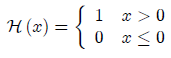Which for a digital call option becomes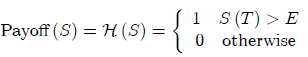The diagram below shows the value (red line) of a digital call at some point in time before expiration, and also illustrates the payoff function (blue line).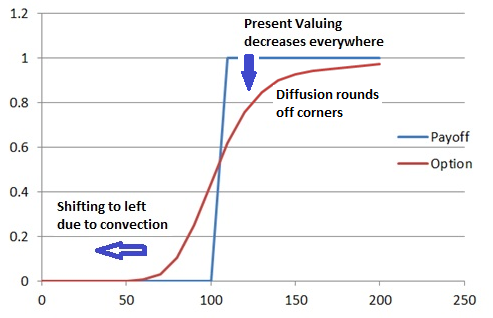Figure 1 - Digital Call Option Payoff vs. Value of Underlying

Each element of the Black-Scholes Equation impacts the shape of the option value.

Discounting from expiration using TVM exerts downward pressure.

: Diffusion term rounds off the corners of the option value graph

:Convection shifts the profile to the left

For a binary option, the Black-Scholes formula is given by: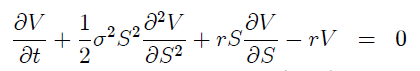The payoff function for the binary call option: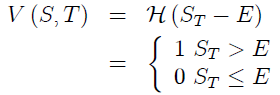S is the spot price of the underlying financial asset, t is the time,
E > 0 is the strike price, T the expiry date, r0 the interest rate and is the volatility of S:

This has a closed-form solution :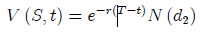Where: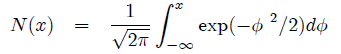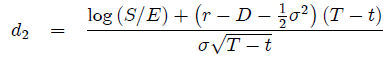The expected value of the discounted payoff under the risk-neutral density is represented by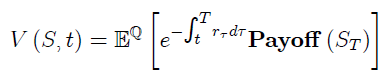An attempt will be made to solve the equation using three methods: (1) Analytical Solution, (2) Explicit Finite Difference Method using backwards marching scheme, and (3) Monte Carlo Simulation using both a Forward Euler-Maruyama and Milstein scheme.

Using the risk-neutral valuation approach, it can be seen

Note the limits on the integral change to x to positive infinity due to the properties of the Heaviside function. If the asset price was below x,the value would be zero and would be zero as well.

Recalling that the asset price follows a lognormal distribution

=

Common Parameters

In performing the calculations, we will use standard inputs into models.

 Option Type: Digital Call Volatility: 20% Expiration 1 (100 timesteps) Risk-free rate: 5% Strike Price: \$100 (initial stock price): \$100
1. Numerical Solution

The solution using Black-Scholes closed form solution is as follows:

 Price of a digital call option under Black Scholes:Given previously: r = 0.05, t = 0, T = 1, E = \$100, = 0.2, = \$100

= = 0.15

N( = N(0.1500) = 0.559618 (using cumulative distribution function for the normal distribution).

* (0.559618) * (0.559618)* (0.559618) = 0.532325

1. Explicit Finite-Difference Method

Finite-difference methods are designed for determining numerical solutions of differential equations using a grid/mesh, similar to the way the Binomial model uses a tree representation. In working with a mesh, the contract value at all points in the stock price-time continuum will be calculated. The Explicit Finite Difference method facilitates the transformation from a differential equation (Black-Scholes) to a difference equation, since its grid/mesh is 'square' and symmetric.

The asset is assumed to follow a lognormal random walk; the interest rate is taken to be as the continuous risk-free rate (following the no-arbitrage principal). The Explicit Finite-Difference valuation method utilizes a risk-neutral valuation approach, common to the Binomial Tree method.

The method is ideally suited to this type of valuation given we are working with a low number (three) of dimensions.

Boundary/Final Conditions
In this numerical scheme, the call defines the final condition at expiration. With this method we start at expiration and work backwards incrementally towards the present. T

The parameters of Finite Difference Method were varied to determine effect on accuracy, increasing the number of asset steps to iteratively refine the answer. The results (included in the spreadsheet submitted with this paper) are shown in Table 1 below .

Algorithm
The code associated with the Explicit Finite-Difference algorithm is included as Appendix "A".

Results
Table 1 and Figure 2 below indicate the option value of the binary call as calculated by the Explicit Finite-Difference method with 10 asset steps: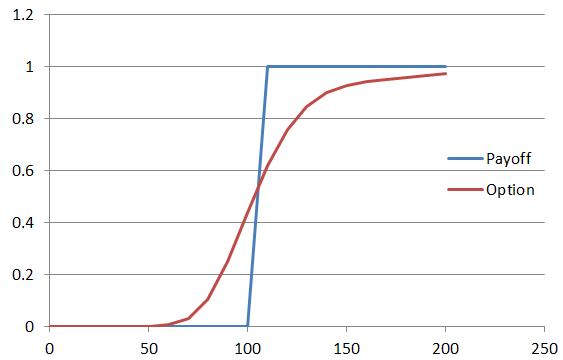Figure 2 - Actual Payoff Function of EFD Method

The numerical experiment was performed using the algorithm to determine how the Explicit Finite-Difference method pricing would contrast against the pricing derived analytically from the Black-Scholes equation. Asset step size was increased from 10 through to 160 using a time period of 1. Figure 3 shows the effect of increasing asset step size on the degree of error, when comparing the EFD numerically derived result against the BSE analytically derived price (\$0.53233). It's clear there is initially an exponential correction in the error rate in increasing asset steps, which then transitions to an approximately linear decay as the number of asset steps continues to increase.

Table 1 - Numerical Results for Cash-or-Nothing digital call option (s=100)

Figure 3 - % Error vs Asset Step Size

1. MONTE CARLO SIMULATION (Forward Euler-Maruyama and Milstein Corrected)

Two types of Monte Carlo simulation were run as part of this investigation. The first type was the regular Forward Euler-Maruyama method, while the second was the Milstein method incorporating a correcting term.

The fair value of an option in the Black-Scholes world is the present value of the expected payoff at expiry under a risk-neutral lognormal random walk for the underlying.

Given the risk-neutral random walk for S is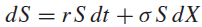We can therefore write:

Provided the expectation is in relation to the risk-neutral random walk, and not the actual one. Therefore the methodology used for MC simulation is:

1. Simulate the risk-neutral random walk as discussed below, starting at today's value of the asset , over the required time horizon. This time period starts today and continues until the expiry of the option. This gives one realization of the underlying price path.
2. For this realization calculate the option payoff.
3. Perform many more such realizations over the time horizon.
4. Calculate the average payoff over all realizations.
5. Take the present value of this average, which results in the derived the option value.
1. Forward Euler-Maruyama Method

The Forward Euler-Maruyama method involves the use of the equation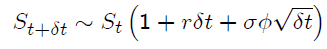Which is based on Geometric Brownian Motion (GBM), specifically the relationship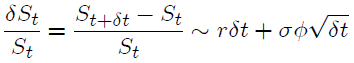The Euler-Maruyama method represents a discrete way of simulating the time series for asset S, and has the advantage of being easy to apply to any stochastic differential equation, irrespective of complexity or order.

The calculation was performed using 16,378 discrete simulations, each representing a unique path through time and a constant =0.01. The number of simulations was increased from 100 to 16,000 while keeping all other variables constant to determine the effect on error.

Results
Table 2 below represents the numerically-derived results from the Monte Carlo simulation using the Forward Euler-Maruyama method. Figure 4 indicates the relationship between error and number of individual simulation paths.

Table 2 - Euler Method Numerical Results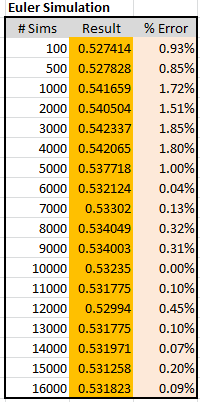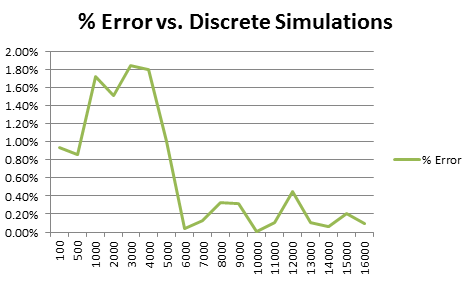Figure 4 - Error vs. Discrete Simulations - Euler Method

1. Milstein Method

The Milstein method of Monte Carlo simulation attempts to increase accuracy through the use of an additional second-order term.

By performing a Taylor series expansion of the exact solution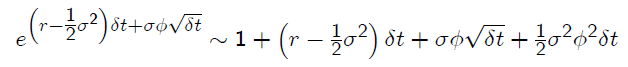The following result is obtained: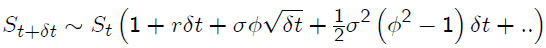This equation differs from the Euler method at by the term (-1). This additional term is referred to as the Milstein correction, and serves as a stochastic effect to reduce simulation error. While the Euler method has an error , the Milstein method's error is reduced to , theoretically making it a more accurate estimator.

Results
Table 2 represents the numerically-derived results from the Monte Carlo simulation using the Milstein method with the correcting stochastic term. Figure 5 indicates the relationship between error and number of individual Milstein simulation paths.

Figure 5 - Error vs. Discrete Simulations: Milstein Method

Table 3 - Milstein Method Numerical Results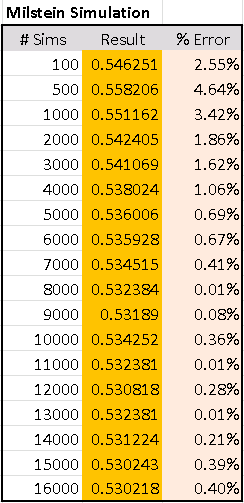1. CONCLUSIONS

The Black-Scholes method is an exact calculation of the option value for a predetermined stream of underlying asset prices. The three analytical solutions investigated here served as the basis for determining their efficiency and accuracy at numerical valuation. In this study we derived the analytical solution for the binary option in the Black-Scholes method and numerical solutions for the Explicit Finite Difference method, the Forward Euler- Maruyama method, and the Milstein method. Examples were provided in all cases for the numerical methods to conclude that all three numerical methods provide valid estimates of the digital call option pricing as compared to the Black-Scholes method.

In the case of the Explicit-Finite Difference method, there was a fairly deterministic relationship between the increase in asset step size and accuracy, and we were able to obtain a result with a slightly less than 2% error factor. The Forward Euler- Maruyama method provided a less predictable result set, although one of the trials (10,000 simulations) produced an exact result to five decimal places - the best of all three numerical methods. Unfortunately, this error reduction did not appear to converge and continued to range between 0.00% and 0.4% as further simulation paths were added. The Milstein method of Monte Carlo simulation produced a similar pattern of error rate vs. number of simulation paths, with the minimum observed percentage error of 0.01% occurring at 8,000 and 13,000 simulation paths. Again, the accuracy ranged between 0.01 and 0.4% beyond 8,000 iterations. This would tend to indicate that the stochastic correction provided by the Milstein method may not correlate with the number of simulation paths within the order of magnitude used in this study. It would be useful to provide a further study with orders of magnitude greater in simulation paths to determine if in fact the Milstein method outperforms its simpler Euler counterpart.

All three methods can be considered as accurate predictors for digital call-option pricing.

Bruce Haydon - Buffalo, New York Copyright Bruce Haydon